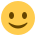#### Howdy, Stranger!

It looks like you're new here. If you want to get involved, click one of these buttons!

Supported by

# Skewness and kurtosis values in descriptives

edited May 2016

Hi all,

I already posted this on Twitter but was referred to the forum, so I'll bring it up here againI recently started using JASP but tend to doublecheck relevant values in R. Today I calculated simple descriptives for my data. In R, I am using the library pastecs, and the values for skewness and kurtosis (as well as the SEs, obviously) differ from those provided in JASP. The general direction is the same (i.e., I have one non-normally distributed variable with high skew and kurtosis), but the numeric values differ quite a bit. All other values are identical though. Any ideas why that might be? Because I am unsure which ones to report now.

• edited 12:43PM

This is how we calculate skewness and kurtosis:

``````.descriptivesKurtosis <- function(x) {

# Kurtosis function as in SPSS:
# http://www.ats.ucla.edu/stat/mult_pkg/faq/general/kurtosis.htm
# http://en.wikipedia.org/wiki/Kurtosis#Estimators_of_population_kurtosis

n <- length(x)
s4 <- sum((x - mean(x))^4)
s2 <- sum((x - mean(x))^2)
v <- s2 / (n-1)
a <- (n * (n + 1)) / ((n - 1) * (n - 2) * (n - 3))
b <- s4 / (v^2)
c <- (-3 * (n - 1)^2) / ((n - 2) * (n - 3))
kurtosis <- a * b + c
return(kurtosis)
}

.descriptivesSkewness <- function(x) {

# Skewness function as in SPSS (for samples spaces):
# http://suite101.com/article/skew-and-how-skewness-is-calculated-in-statistical-software-a231005

n <- length(x)
m <- mean(x)
s <- sd(x)
z <- (x - m) / s  # z scores
a <- n / ((n - 1) * (n - 2))
skewness <- sum(z^3) * a
return(skewness)
}
``````

I'm not sure how the R package calculates these statistics. Could you attach the csv you are using to calculate these statistics so I can check it?